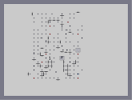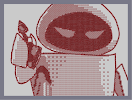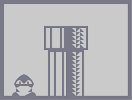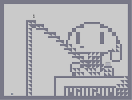### WOO-HOO!Hover over the thumbnail for a full-size version.

Author darkcrusader author:darkcrusader darkcrusader gold n-art nart nonplayable rated 2010-09-05 2010-09-05 5 by 6 people. \$WOO-HOO!#darkcrusader#nonplayable#0000000000000000000000000000000000000000000000000000000000000000000000000000000000000000000000000000000000000000000000000000000000000000000000000000000000000000000000000000000000000000000000000000000000000000000000000000000000000000000000000000000000000000000000000000000000000000000000000000000000000000000000000000000000000000000000000000000000000000000000000000000000000000000000000000000000000000000000000000000000000000000000000000000000000000000QQP00000000000000000000QQP00000000000000000000QQ000000000000000000000NQP00000000000000000000NOP0000000000000000000000000000000000000000000NQP00000000000000000000NOP00000000000000000000000000000000000000000001PP0000000000000000000000000000000000000000000000000000|12^468,84!12^480,552!12^384,300!12^456,540!12^468,576!12^432,576!12^444,90!12^396,186!12^396,390!12^384,246!12^439,515!12^404,430!12^390,356!12^385,325!12^389,213!12^408,155!12^432,104!12^452,89!12^455,88!12^465,85!12^447,89!12^443,90!12^441,94!12^437,98!12^435,100!12^431,106!12^428,111!12^426,117!12^424,121!12^423,123!12^415,138!12^418,133!12^413,142!12^411,146!12^409,150!12^406,161!12^403,166!12^402,171!12^400,179!12^398,185!12^401,175!12^394,193!12^392,199!12^391,205!12^387,219!12^387,225!12^385,232!12^386,236!12^385,239!12^385,243!12^384,276!12^383,269!12^383,263!12^383,256!12^384,246!12^383,255!12^384,281!12^385,249!12^386,230!12^390,209!12^421,129!12^385,324!12^385,319!12^385,312!12^384,305!12^384,299!12^384,295!12^384,291!12^384,286!12^386,331!12^388,336!12^389,343!12^389,351!12^388,349!12^385,316!12^384,311!12^384,277!12^384,260!12^394,386!12^394,380!12^390,361!12^390,367!12^392,370!12^394,376!12^404,427!12^402,425!12^401,420!12^400,417!12^400,415!12^400,411!12^399,410!12^399,405!12^398,405!12^398,402!12^397,400!12^397,393!12^417,469!12^413,459!12^412,455!12^410,451!12^409,446!12^408,440!12^406,435!12^406,430!12^439,513!12^436,507!12^433,503!12^433,502!12^431,497!12^428,493!12^427,489!12^420,474!12^419,472!12^416,468!12^415,465!12^425,488!12^424,486!12^424,484!12^421,479!12^454,537!12^451,533!12^450,531!12^447,528!12^447,524!12^445,523!12^443,520!12^476,552!12^472,549!12^467,547!12^464,546!12^460,544!12^478,557!12^476,562!12^474,566!12^471,568!12^471,569!12^469,573!12^474,555!12^470,558!12^468,561!12^466,562!12^464,566!12^464,572!12^467,573!12^462,576!12^456,576!12^450,576!12^444,576!12^438,576!12^444,576!12^468,84!12^472,81!12^473,77!12^474,72!12^475,66!12^474,61!12^470,57!12^467,56!12^466,57!12^465,60!12^464,65!12^466,71!12^470,76!12^463,54!12^447,55!12^459,55!12^453,55!12^449,54!12^444,55!12^304,529!12^403,571!12^429,574!12^424,574!12^417,574!12^413,573!12^406,573!12^350,556!12^209,219!12^251,141!12^382,60!12^401,58!12^398,58!12^392,59!12^388,59!12^376,61!12^369,61!12^366,63!12^330,69!12^335,67!12^339,65!12^346,65!12^351,63!12^358,63!12^362,62!12^366,62!12^268,119!12^278,108!12^280,106!12^283,103!12^275,111!12^271,116!12^265,122!12^262,126!12^253,138!12^255,134!12^257,131!12^260,128!12^276,113!12^226,176!12^247,146!12^233,163!12^216,197!12^200,259!12^201,344!12^225,426!12^277,508!12^326,545!12^378,567!12^393,571!12^396,572!12^213,207!12^220,185!12^229,170!12^236,158!12^241,152!12^223,180!12^219,192!12^214,201!12^211,213!12^208,225!12^206,230!12^205,234!12^202,245!12^202,252!12^201,254!12^198,302!12^198,290!12^199,264!12^199,286!12^199,279!12^199,273!12^199,269!12^199,297!12^199,305!12^200,328!12^202,340!12^201,337!12^201,334!12^200,325!12^200,323!12^200,319!12^200,316!12^199,315!12^198,312!12^206,367!12^203,358!12^202,351!12^203,360!12^203,239!12^246,146!12^305,84!12^328,71!12^319,75!12^314,77!12^310,81!12^323,73!12^288,101!12^292,96!12^297,92!12^303,87!12^304,85!12^364,563!12^265,492!12^291,520!12^316,537!12^336,550!12^232,438!12^218,408!12^212,391!12^208,377!12^207,372!12^211,388!12^209,383!12^218,407!12^217,402!12^215,396!12^225,424!12^223,422!12^222,417!12^220,413!12^231,434!12^230,432!12^230,431!12^235,443!12^262,489!12^245,463!12^239,452!12^236,447!12^243,460!12^241,457!12^247,467!12^259,485!12^257,481!12^257,480!12^254,476!12^252,474!12^249,472!12^276,505!12^274,504!12^271,502!12^270,499!12^266,496!12^289,519!12^286,518!12^286,515!12^283,512!12^281,512!12^301,527!12^297,525!12^296,524!12^314,535!12^310,533!12^324,543!12^319,542!12^319,540!12^334,550!12^351,556!12^347,555!12^342,553!12^340,552!12^331,548!12^389,571!12^386,571!12^383,568!12^377,568!12^372,567!12^368,565!12^360,562!12^353,558!12^358,561!0^468,552!0^462,558!0^456,564!0^456,570!0^450,570!0^450,564!0^456,558!0^462,552!0^456,552!0^450,558!0^444,564!0^444,570!0^438,570!0^438,564!0^438,558!0^444,558!0^444,552!0^450,552!0^456,546!0^450,546!0^444,546!0^438,552!0^438,546!0^444,540!0^450,540!0^438,540!0^438,534!0^444,534!0^438,528!0^438,522!0^432,570!0^426,570!0^420,570!0^420,570!0^414,570!0^408,570!0^402,570!0^396,564!0^390,564!0^402,564!0^408,564!0^420,564!0^414,564!0^426,564!0^432,564!0^384,564!0^378,564!0^372,558!0^366,558!0^360,558!0^378,558!0^384,558!12^379,567!12^360,561!0^432,522!0^432,516!0^432,510!0^426,510!0^426,504!0^426,498!0^420,498!0^420,492!0^414,486!0^414,474!0^414,480!0^408,474!0^408,468!0^408,462!0^402,450!0^402,456!0^402,444!0^396,432!0^396,438!0^396,426!0^390,426!0^390,420!0^390,414!0^390,408!0^390,402!0^390,396!0^384,396!0^384,390!0^384,384!0^384,378!0^384,372!0^384,366!0^384,354!0^384,360!0^384,348!0^384,342!0^378,342!0^378,336!0^378,330!0^378,324!0^378,318!0^378,312!0^378,306!0^378,306!0^378,300!0^378,294!0^378,288!0^378,276!0^378,282!0^378,270!0^378,264!0^378,258!0^378,252!0^378,246!0^378,240!0^378,234!0^378,222!0^378,228!0^384,216!0^384,204!0^384,210!0^384,198!0^384,192!0^390,186!0^396,180!0^396,174!0^396,168!0^402,156!0^396,162!0^402,150!0^402,138!0^402,144!0^408,138!0^408,132!0^414,132!0^414,126!0^414,120!0^420,114!0^420,108!0^420,96!0^420,102!0^426,96!0^432,96!0^432,90!0^438,84!0^426,84!0^432,84!0^426,90!0^444,84!0^450,84!0^462,78!0^456,84!12^462,88!0^456,72!0^456,66!0^456,60!0^450,60!0^444,60!12^438,54!12^432,54!12^426,54!12^406,57!12^413,55!12^418,55!12^422,54!0^438,60!0^432,60!0^426,60!0^420,60!0^414,60!0^408,60!0^408,66!0^402,66!0^396,66!0^384,66!0^390,66!0^378,66!0^372,66!0^372,72!0^366,72!0^360,72!0^354,72!0^342,72!0^348,72!0^336,78!0^330,78!0^330,84!0^324,84!0^318,84!0^318,90!0^312,90!0^312,96!0^306,96!0^306,102!0^300,102!0^294,102!0^294,108!0^288,108!0^288,114!0^282,120!0^294,120!0^288,126!0^288,120!0^282,126!0^276,126!0^276,120!0^282,114!0^270,126!0^270,132!0^264,132!0^264,138!0^258,138!0^258,144!0^258,150!0^252,150!0^246,156!0^252,156!0^246,162!0^240,162!0^240,168!0^240,174!0^234,174!0^234,180!0^234,186!0^228,186!0^228,192!0^222,198!0^222,210!0^222,210!0^222,204!0^216,222!0^222,216!0^216,216!0^216,228!0^216,234!0^216,240!0^210,246!0^210,240!0^210,252!0^210,258!0^210,240!0^210,234!0^216,210!0^228,180!0^234,168!12^231,168!0^390,390!0^402,438!0^384,222!0^426,102!0^456,78!0^204,270!0^204,264!0^204,276!0^204,288!0^204,282!0^204,294!0^204,306!0^204,300!0^204,312!0^204,318!0^204,324!0^204,330!0^204,336!0^204,342!0^210,348!0^210,354!0^210,360!0^210,366!0^210,372!0^216,372!0^216,378!0^216,384!0^216,390!0^222,390!0^222,402!0^222,396!0^222,408!0^228,408!0^228,414!0^228,420!0^234,426!0^234,420!0^234,432!0^240,432!0^240,438!0^240,444!0^360,552!0^354,552!0^354,546!0^348,546!0^342,546!0^336,546!0^336,540!0^330,540!0^330,534!0^324,534!0^324,528!0^318,528!0^312,528!0^312,522!0^306,522!0^306,522!0^300,522!0^300,516!0^294,510!0^288,510!0^282,504!0^276,498!0^270,492!0^270,492!0^270,486!0^264,486!0^264,480!0^258,474!0^258,468!0^252,462!0^246,450!0^246,456!0^252,468!12^334,549!12^299,528!12^297,528!0^246,264!0^246,270!0^252,276!0^252,282!0^252,288!0^258,270!0^258,264!0^270,288!0^270,276!0^270,282!0^270,270!0^276,264!0^282,270!0^282,276!0^282,282!0^282,288!0^276,276!0^294,264!0^294,270!0^300,276!0^300,282!0^300,288!0^306,270!0^306,264!0^318,288!0^324,288!0^324,294!0^318,300!0^246,318!0^240,318!0^234,324!0^234,330!0^234,336!0^240,342!0^246,342!0^258,342!0^258,336!0^258,330!0^258,324!0^264,318!0^270,324!0^270,330!0^270,336!0^270,342!0^264,330!0^282,342!0^282,336!0^282,330!0^282,324!0^282,318!0^288,330!0^294,324!0^294,318!0^294,336!0^294,342!0^306,318!0^306,330!0^306,324!0^306,336!0^306,342!0^312,342!0^318,342!0^312,330!0^312,318!0^318,318!0^330,318!0^330,324!0^330,330!0^330,342!0^450,78!0^444,72!0^450,72!0^450,66!0^444,66!0^444,78!0^438,78!0^432,78!0^432,72!0^438,72!0^438,66!0^432,66!0^426,78!0^426,72!0^426,66!0^420,90!0^420,84!0^420,78!0^420,72!0^420,66!0^414,66!0^414,72!0^408,72!0^402,72!0^396,72!0^390,72!0^384,72!0^378,72!0^414,78!0^414,84!0^414,90!0^414,96!0^414,102!0^414,108!0^414,114!0^408,78!0^402,78!0^396,78!0^390,78!0^384,78!0^378,78!0^372,78!0^366,78!0^360,78!0^354,78!0^348,78!0^342,78!0^408,84!0^408,90!0^408,96!0^408,102!0^408,108!0^408,114!0^408,120!0^408,126!0^402,84!0^396,84!0^390,84!0^384,84!0^378,84!0^372,84!0^366,84!0^360,84!0^354,84!0^348,84!0^342,84!0^336,84!0^408,480!0^408,492!0^408,486!0^408,504!0^408,498!0^408,510!0^408,516!0^408,522!0^408,534!0^408,528!0^408,540!0^408,546!0^408,552!0^408,558!0^414,558!0^420,558!0^426,558!0^432,558!0^432,552!0^420,552!0^426,552!0^420,552!0^414,552!0^414,546!0^420,546!0^426,546!0^432,546!0^432,540!0^426,540!0^420,540!0^414,540!0^414,534!0^420,534!0^426,534!0^432,534!0^432,528!0^426,528!0^426,522!0^426,516!0^420,522!0^420,528!0^414,528!0^414,522!0^414,516!0^420,516!0^420,510!0^414,510!0^414,504!0^420,504!0^414,498!0^414,492!0^402,90!0^402,102!0^402,96!0^402,114!0^402,108!0^402,120!0^402,126!0^402,138!0^402,132!0^402,462!0^402,468!0^402,474!0^402,480!0^402,492!0^402,486!0^402,504!0^402,498!0^402,510!0^402,516!0^402,522!0^402,528!0^402,534!0^402,540!0^402,546!0^402,552!0^402,558!0^396,90!0^390,90!0^384,90!0^378,90!0^372,90!0^372,90!0^366,90!0^360,90!0^354,90!0^348,90!0^342,90!0^336,90!0^330,90!0^324,90!0^396,96!0^396,102!0^396,108!0^396,114!0^396,120!0^396,126!0^396,132!0^396,138!0^396,144!0^396,150!0^396,156!0^396,444!0^396,450!0^396,456!0^396,462!0^396,468!0^396,474!0^396,480!0^396,486!0^396,492!0^396,504!0^396,498!0^396,510!0^396,516!0^396,522!0^396,528!0^396,534!0^396,540!0^396,546!0^396,552!0^396,558!0^390,96!0^384,96!0^378,96!0^372,96!0^366,96!0^360,96!0^354,96!0^348,96!0^342,96!0^336,96!0^330,96!0^324,96!0^318,96!0^390,102!0^390,108!0^390,120!0^390,114!0^390,126!0^390,132!0^390,138!0^390,144!0^390,150!0^390,156!0^390,162!0^390,168!0^390,174!0^390,180!0^390,432!0^390,438!0^390,444!0^390,450!0^390,462!0^390,456!0^390,474!0^390,468!0^390,480!0^390,486!0^390,492!0^390,498!0^390,504!0^390,510!0^390,516!0^390,522!0^390,534!0^390,528!0^390,540!0^390,546!0^390,552!0^390,558!0^384,102!0^378,102!0^372,102!0^366,102!0^360,102!0^354,102!0^348,102!0^342,102!0^336,102!0^330,102!0^324,102!0^318,102!0^312,102!0^378,108!0^384,108!0^384,114!0^384,120!0^384,126!0^384,132!0^384,138!0^384,144!0^384,150!0^384,156!0^384,162!0^384,168!0^384,180!0^384,186!0^384,174!0^384,402!0^384,408!0^384,414!0^384,420!0^384,426!0^384,432!0^384,438!0^384,444!0^384,450!0^384,456!0^384,462!0^384,474!0^384,468!0^384,480!0^384,486!0^384,492!0^384,498!0^384,504!0^384,510!0^384,516!0^384,522!0^384,528!0^384,534!0^384,540!0^384,546!0^384,552!0^372,108!0^366,108!0^360,108!0^354,108!0^348,108!0^342,108!0^336,108!0^324,108!0^330,108!0^318,108!0^312,108!0^306,108!0^300,108!0^378,114!0^378,120!0^378,126!0^378,132!0^378,138!0^378,144!0^378,150!0^378,156!0^378,162!0^378,168!0^378,174!0^378,180!0^378,186!0^378,192!0^378,198!0^378,204!0^378,210!0^378,216!0^378,348!0^378,354!0^378,360!0^378,366!0^378,372!0^378,378!0^378,384!0^378,390!0^378,396!0^378,402!0^378,408!0^378,414!0^378,420!0^378,426!0^378,432!0^378,438!0^378,444!0^378,450!0^378,456!0^378,462!0^378,468!0^378,474!0^378,480!0^378,486!0^378,492!0^378,498!0^378,504!0^378,510!0^378,516!0^378,522!0^378,528!0^378,534!0^378,540!0^378,546!0^378,552!0^372,114!0^366,114!0^360,114!0^354,114!0^348,114!0^342,114!0^336,114!0^330,114!0^324,114!0^318,114!0^312,114!0^306,114!0^300,114!0^294,114!0^372,120!0^372,126!0^372,132!0^372,138!0^372,144!0^372,150!0^372,156!0^372,162!0^372,168!0^372,174!0^372,180!0^372,186!0^372,192!0^372,198!0^372,204!0^372,210!0^372,216!0^372,222!0^372,228!0^372,234!0^372,240!0^372,246!0^372,252!0^372,258!0^372,264!0^372,276!0^372,270!0^372,282!0^372,294!0^372,288!0^372,300!0^372,306!0^372,312!0^372,318!0^372,324!0^372,330!0^372,336!0^372,342!0^372,348!0^372,354!0^372,360!0^372,366!0^372,372!0^372,378!0^372,384!0^372,390!0^372,396!0^372,402!0^372,408!0^372,414!0^372,420!0^372,426!0^372,432!0^372,438!0^372,444!0^372,450!0^372,456!0^372,462!0^372,468!0^372,474!0^372,480!0^372,486!0^372,492!0^372,498!0^372,504!0^372,510!0^372,516!0^372,522!0^372,528!0^372,534!0^372,540!0^372,546!0^372,552!0^366,120!0^360,120!0^354,120!0^348,120!0^342,120!0^336,114!0^336,120!0^330,120!0^324,120!0^318,120!0^312,120!0^306,120!0^300,120!0^366,126!0^366,132!0^366,138!0^366,144!0^366,150!0^366,156!0^366,162!0^366,168!0^366,174!0^366,180!0^366,186!0^366,192!0^366,198!0^366,204!0^366,210!0^366,216!0^366,222!0^366,228!0^366,234!0^366,240!0^366,246!0^366,252!0^366,258!0^366,264!0^366,270!0^366,276!0^366,282!0^366,288!0^366,294!0^366,300!0^366,306!0^366,312!0^366,318!0^366,324!0^366,330!0^366,336!0^366,342!0^366,348!0^366,354!0^366,360!0^366,366!0^366,372!0^366,378!0^366,384!0^366,390!0^366,396!0^366,402!0^366,408!0^366,414!0^366,420!0^366,426!0^366,432!0^366,438!0^366,444!0^366,450!0^366,456!0^366,462!0^366,468!0^366,474!0^366,480!0^366,486!0^366,492!0^366,498!0^366,504!0^366,510!0^366,516!0^366,522!0^366,528!0^366,534!0^366,540!0^366,546!0^366,552!0^360,126!0^354,126!0^348,126!0^342,126!0^330,126!0^336,126!0^324,126!0^318,126!0^312,126!0^306,126!0^300,126!0^294,126!0^360,132!0^360,138!0^360,144!0^360,150!0^360,156!0^360,162!0^360,168!0^360,174!0^360,180!0^360,186!0^360,192!0^360,198!0^360,204!0^360,210!0^360,216!0^360,222!0^360,228!0^360,234!0^360,240!0^360,246!0^360,252!0^360,258!0^360,264!0^360,270!0^360,276!0^360,282!0^360,288!0^360,294!0^360,300!0^360,306!0^360,312!0^360,318!0^360,324!0^360,330!0^360,336!0^360,342!0^360,348!0^360,354!0^360,360!0^360,366!0^360,366!0^360,372!0^360,378!0^360,384!0^360,390!0^360,396!0^360,402!0^360,408!0^360,414!0^360,420!0^360,420!0^360,426!0^360,432!0^360,438!0^360,444!0^360,456!0^360,450!0^360,462!0^360,474!0^360,468!0^360,480!0^360,486!0^360,492!0^360,498!0^360,510!0^360,504!0^360,516!0^366,522!0^360,522!0^360,528!0^360,534!0^360,540!0^360,546!0^354,132!0^348,132!0^342,132!0^336,132!0^330,132!0^324,132!0^318,132!0^312,132!0^306,132!0^300,132!0^294,132!0^288,132!0^282,132!0^276,132!0^354,138!0^354,144!0^354,150!0^354,156!0^354,162!0^354,168!0^354,174!0^354,180!0^354,186!0^354,192!0^354,198!0^354,204!0^354,210!0^354,216!0^354,222!0^354,228!0^354,234!0^354,240!0^354,246!0^354,252!0^354,258!0^354,264!0^354,270!0^354,276!0^354,282!0^354,288!0^354,294!0^354,312!0^354,318!0^354,324!0^354,336!0^354,342!0^354,330!0^354,348!0^354,354!0^354,360!0^354,372!0^354,378!0^354,366!0^354,384!0^354,390!0^354,396!0^354,402!0^354,408!0^354,414!0^354,420!0^354,426!0^360,432!0^354,432!0^354,444!0^354,438!0^354,450!0^354,456!0^354,462!0^354,468!0^354,474!0^354,480!0^354,486!0^354,492!0^354,498!0^354,504!0^354,510!0^354,516!0^354,522!0^354,528!0^354,534!0^354,540!0^348,138!0^342,138!0^336,138!0^330,138!0^324,138!0^318,138!0^312,138!0^306,138!0^300,138!0^294,138!0^288,138!0^282,138!0^276,138!0^270,138!0^348,144!0^348,150!0^348,156!0^348,162!0^348,168!0^348,174!0^348,186!0^348,180!0^348,192!0^348,198!0^348,204!0^348,210!0^348,216!0^348,222!0^348,228!0^348,234!0^348,240!0^348,246!0^348,252!0^348,258!0^348,264!0^348,270!0^348,276!0^348,282!0^348,288!0^348,294!0^348,312!0^348,318!0^348,324!0^348,330!0^348,336!0^348,348!0^348,354!0^348,360!0^348,366!0^348,372!0^348,378!0^348,384!0^348,390!0^348,396!0^348,402!0^348,408!0^348,414!0^348,420!0^348,426!0^348,432!0^348,438!0^348,444!0^348,450!0^348,456!0^348,462!0^348,468!0^348,474!0^348,480!0^348,486!0^348,492!0^348,498!0^348,504!0^348,510!0^348,516!0^348,522!0^348,528!0^348,534!0^348,540!0^342,144!0^336,144!0^330,144!0^324,144!0^318,144!0^312,144!0^306,144!0^300,144!0^294,144!0^288,138!0^288,144!0^282,144!0^276,144!0^270,144!0^264,144!0^342,150!0^342,156!0^342,162!0^342,168!0^342,174!0^342,180!0^342,186!0^342,192!0^342,198!0^342,204!0^342,210!0^342,216!0^342,222!0^342,234!0^342,228!0^342,240!0^342,246!0^342,252!0^342,258!0^342,264!0^342,270!0^342,276!0^342,282!0^342,288!0^342,294!0^342,300!0^342,306!0^342,312!0^342,318!0^342,324!0^342,330!0^342,336!0^342,342!0^342,348!0^342,354!0^342,360!0^342,366!0^342,372!0^342,378!0^342,384!0^342,390!0^348,396!0^342,396!0^342,402!0^342,408!0^342,414!0^342,420!0^342,426!0^342,432!0^342,438!0^342,444!0^342,450!0^342,456!0^342,468!0^342,462!0^342,474!0^342,480!0^342,486!0^342,492!0^342,498!0^342,504!0^342,510!0^342,516!0^342,522!0^342,528!0^342,534!0^342,540!0^306,150!0^336,150!0^330,150!0^324,150!0^318,150!0^312,150!0^300,150!0^294,150!0^288,150!0^282,150!0^276,150!0^270,150!0^264,150!0^336,156!0^336,162!0^336,168!0^336,174!0^336,180!0^336,186!0^336,192!0^336,198!0^336,204!0^336,210!0^336,216!0^336,222!0^336,228!0^336,234!0^336,240!0^336,246!0^336,252!0^336,258!0^336,264!0^336,270!0^336,276!0^336,282!0^336,288!0^336,294!0^336,300!0^336,306!0^336,312!0^336,318!0^336,324!0^336,330!0^336,336!0^336,342!0^336,348!0^336,354!0^336,360!0^336,366!0^336,372!0^336,378!0^336,390!0^336,384!0^336,396!0^336,402!0^336,408!0^336,414!0^336,420!0^336,426!0^336,432!0^336,438!0^336,444!0^336,450!0^336,456!0^336,462!0^336,468!0^336,474!0^336,480!0^336,486!0^336,492!0^336,498!0^336,504!0^336,510!0^336,516!0^336,522!0^336,528!0^336,534!0^330,156!0^324,156!0^318,156!0^312,156!0^306,156!0^300,156!0^294,156!0^288,156!0^282,156!0^276,156!0^270,156!0^264,156!0^258,156!0^330,162!0^330,168!0^330,174!0^330,180!0^330,186!0^330,192!0^330,198!0^330,204!0^330,210!0^330,216!0^330,222!0^330,228!0^330,234!0^330,240!0^330,246!0^330,252!0^330,258!0^330,264!0^330,270!0^330,276!0^330,282!0^330,288!0^330,294!0^330,300!0^330,306!0^330,312!0^330,336!0^330,348!0^330,354!0^330,360!0^330,366!0^330,372!0^330,378!0^330,384!0^330,390!0^330,396!0^330,402!0^330,408!0^330,414!0^330,420!0^330,426!0^330,432!0^330,438!0^330,444!0^330,450!0^330,456!0^330,462!0^330,468!0^330,474!0^330,480!0^330,486!0^330,492!0^330,498!0^330,504!0^330,510!0^330,516!0^330,522!0^330,528!0^324,162!0^318,162!0^312,162!0^306,162!0^300,162!0^294,162!0^288,162!0^276,162!0^282,162!0^270,168!0^264,162!0^270,162!0^258,162!0^252,162!0^324,162!0^324,168!0^318,168!0^312,168!0^300,168!0^306,168!0^294,168!0^288,168!0^282,168!0^276,168!0^264,168!0^258,168!0^252,168!0^246,168!0^324,174!0^324,180!0^324,186!0^324,192!0^324,198!0^324,204!0^324,210!0^324,216!0^324,222!0^324,228!0^324,234!0^324,240!0^324,246!0^324,252!0^324,258!0^324,264!0^324,270!0^324,276!0^324,282!0^324,300!0^324,306!0^324,312!0^324,318!0^324,324!0^324,330!0^324,336!0^324,342!0^324,348!0^324,354!0^324,360!0^324,366!0^324,372!0^324,378!0^324,384!0^324,390!0^324,396!0^324,402!0^324,408!0^324,414!0^324,420!0^324,426!0^324,432!0^324,438!0^324,444!0^324,450!0^324,456!0^324,462!0^324,468!0^324,474!0^324,480!0^324,486!0^324,492!0^324,498!0^324,504!0^324,510!0^324,516!0^324,522!0^318,174!0^312,174!0^306,174!0^300,174!0^294,174!0^288,174!0^282,174!0^276,174!0^270,174!0^264,174!0^258,174!0^246,174!0^252,174!0^318,180!0^318,192!0^318,186!0^318,198!0^318,204!0^318,210!0^318,216!0^318,222!0^318,228!0^318,234!0^318,240!0^318,246!0^318,252!0^318,258!0^318,270!0^318,264!0^318,276!0^318,282!0^318,294!0^318,306!0^318,312!0^318,324!0^318,330!0^318,336!0^318,348!0^318,354!0^318,366!0^318,360!0^318,372!0^318,384!0^318,378!0^318,390!0^318,402!0^318,396!0^318,408!0^318,414!0^318,420!0^318,432!0^318,426!0^318,438!0^318,444!0^318,450!0^318,456!0^318,462!0^318,468!0^318,474!0^318,486!0^318,480!0^318,492!0^318,498!0^318,504!0^318,510!0^318,516!0^318,522!0^312,180!0^306,180!0^300,180!0^294,180!0^288,180!0^282,180!0^276,180!0^270,180!0^264,180!0^258,180!0^252,180!0^246,180!0^240,180!0^312,186!0^312,192!0^312,198!0^312,204!0^312,210!0^312,222!0^312,216!0^312,228!0^312,234!0^312,240!0^312,246!0^312,252!0^312,258!0^312,270!0^312,264!0^312,282!0^312,276!0^312,294!0^312,288!0^312,306!0^312,312!0^312,300!0^312,324!0^312,336!0^312,348!0^312,354!0^312,360!0^312,366!0^312,372!0^312,378!0^312,384!0^312,390!0^312,396!0^312,402!0^312,408!0^312,414!0^312,420!0^312,426!0^312,438!0^312,432!0^312,444!0^312,450!0^312,462!0^312,462!0^312,456!0^312,468!0^312,474!0^312,480!0^312,486!0^312,492!0^312,504!0^312,498!0^312,510!0^312,522!0^312,516!0^306,186!0^300,186!0^294,186!0^282,186!0^288,186!0^276,186!0^270,186!0^264,186!0^258,186!0^252,186!0^246,186!0^240,186!0^306,192!0^306,198!0^306,204!0^306,210!0^306,216!0^306,222!0^306,228!0^306,234!0^306,240!0^306,246!0^306,252!0^306,258!0^306,276!0^306,282!0^306,288!0^306,294!0^306,300!0^306,306!0^306,312!0^306,348!0^306,354!0^306,360!0^306,366!0^306,378!0^306,372!0^306,384!0^306,390!0^306,396!0^306,402!0^306,408!0^306,414!0^306,420!0^306,426!0^306,432!0^306,438!0^306,444!0^306,450!0^306,456!0^306,462!0^306,468!0^306,474!0^306,480!0^312,486!0^306,486!0^306,492!0^306,498!0^306,504!0^306,510!0^306,516!0^300,192!0^294,192!0^288,192!0^282,192!0^276,192!0^270,192!0^264,192!0^258,192!0^252,192!0^246,192!0^240,192!0^234,192!0^300,198!0^300,204!0^300,210!0^300,216!0^300,222!0^300,228!0^300,234!0^300,240!0^300,246!0^300,252!0^300,252!0^300,258!0^306,264!0^300,264!0^300,270!0^300,294!0^300,300!0^300,306!0^300,312!0^300,324!0^300,318!0^300,330!0^300,336!0^300,342!0^300,348!0^300,354!0^300,360!0^300,366!0^300,372!0^300,378!0^300,384!0^300,390!0^300,396!0^300,402!0^300,408!0^300,414!0^300,420!0^300,426!0^300,432!0^300,438!0^300,438!0^300,444!0^300,450!0^300,456!0^300,468!0^300,462!0^300,474!0^300,480!0^300,486!0^300,492!0^300,498!0^300,504!0^300,510!0^294,198!0^288,198!0^282,198!0^276,198!0^270,198!0^264,198!0^258,198!0^252,198!0^246,198!0^240,198!0^234,198!0^228,198!0^294,204!0^294,210!0^294,216!0^294,222!0^294,228!0^294,234!0^294,240!0^294,246!0^294,252!0^294,258!0^294,276!0^294,282!0^294,288!0^294,294!0^294,300!0^294,306!0^294,312!0^294,330!0^294,348!0^294,354!0^294,360!0^294,366!0^294,372!0^294,378!0^294,384!0^294,390!0^294,396!0^294,402!0^294,408!0^294,414!0^294,420!0^294,426!0^294,432!0^294,438!0^294,444!0^294,450!0^294,456!0^294,462!0^294,468!0^294,480!0^294,474!0^294,486!0^294,492!0^294,492!0^294,498!0^294,504!0^288,204!0^282,204!0^276,204!0^270,204!0^264,204!0^258,204!0^258,204!0^246,204!0^252,204!0^240,204!0^234,204!0^228,204!0^288,210!0^288,216!0^288,222!0^288,228!0^288,234!0^288,240!0^288,246!0^288,252!0^288,258!0^288,264!0^288,270!0^288,276!0^288,282!0^288,288!0^288,294!0^288,300!0^288,306!0^288,312!0^288,318!0^288,324!0^288,336!0^288,342!0^288,348!0^288,360!0^288,354!0^288,366!0^288,372!0^288,378!0^288,384!0^288,390!0^288,396!0^282,402!0^288,402!0^288,408!0^288,414!0^288,420!0^288,426!0^288,432!0^288,438!0^288,444!0^288,450!0^288,456!0^288,462!0^288,468!0^288,474!0^288,480!0^288,486!0^288,492!0^288,498!0^288,504!0^282,210!0^276,210!0^270,210!0^264,210!0^258,210!0^252,210!0^246,210!0^240,210!0^234,210!0^228,210!0^282,216!0^276,216!0^270,216!0^264,216!0^258,216!0^252,216!0^246,216!0^240,216!0^234,216!0^228,216!0^282,222!0^282,228!0^282,234!0^282,240!0^282,246!0^282,252!0^282,258!0^282,264!0^282,294!0^282,300!0^282,306!0^282,312!0^282,348!0^282,360!0^282,354!0^282,372!0^282,366!0^282,378!0^282,384!0^282,390!0^282,396!0^282,408!0^282,414!0^282,420!0^282,426!0^282,432!0^282,438!0^282,450!0^282,444!0^282,456!0^282,462!0^282,468!0^282,474!0^282,480!0^282,486!0^282,492!0^282,498!0^276,222!0^270,228!0^270,222!0^264,222!0^258,222!0^252,222!0^246,222!0^240,222!0^234,222!0^228,222!0^222,222!0^276,228!0^276,234!0^276,240!0^276,246!0^276,252!0^276,258!0^276,264!0^276,270!0^276,282!0^276,288!0^276,300!0^276,306!0^276,294!0^276,312!0^276,318!0^276,324!0^276,330!0^276,336!0^276,342!0^276,348!0^276,354!0^276,360!0^276,366!0^276,372!0^276,378!0^276,384!0^276,390!0^276,396!0^276,402!0^270,408!0^276,408!0^276,414!0^276,420!0^276,426!0^276,432!0^276,438!0^276,444!0^276,450!0^276,456!0^276,462!0^276,468!0^276,480!0^276,474!0^276,486!0^276,492!0^294,516!0^366,558!0^264,228!0^258,234!0^258,228!0^252,228!0^246,228!0^240,228!0^234,228!0^228,228!0^222,228!0^270,234!0^270,246!0^270,240!0^270,252!0^270,258!0^270,264!0^270,294!0^270,300!0^270,306!0^270,312!0^270,324!0^270,318!0^270,348!0^270,354!0^270,360!0^270,366!0^270,372!0^270,378!0^270,390!0^270,384!0^270,396!0^270,402!0^270,414!0^270,420!0^270,426!0^270,432!0^270,438!0^270,444!0^270,450!0^270,456!0^270,462!0^270,468!0^264,474!0^264,480!0^270,480!0^270,474!0^264,234!0^252,234!0^246,234!0^240,234!0^234,234!0^228,234!0^222,234!0^264,240!0^264,246!0^264,258!0^264,252!0^264,270!0^264,276!0^264,264!0^264,282!0^264,288!0^264,294!0^264,300!0^264,306!0^264,312!0^264,324!0^264,342!0^264,336!0^264,348!0^264,354!0^264,360!0^264,366!0^264,372!0^264,378!0^264,384!0^264,390!0^264,396!0^264,402!0^264,408!0^264,420!0^264,414!0^264,432!0^264,426!0^264,438!0^264,450!0^264,444!0^264,456!0^264,462!0^264,468!0^264,474!0^258,240!0^252,240!0^246,240!0^240,240!0^234,240!0^228,240!0^222,240!0^258,246!0^258,252!0^258,264!0^258,258!0^258,276!0^258,282!0^258,288!0^258,294!0^258,300!0^258,312!0^258,306!0^258,318!0^258,348!0^258,354!0^258,366!0^258,360!0^258,372!0^258,378!0^258,384!0^258,390!0^258,396!0^258,402!0^258,408!0^258,414!0^258,420!0^258,426!0^246,426!0^258,432!0^258,438!0^258,444!0^258,450!0^258,456!0^258,462!0^252,246!0^246,246!0^240,246!0^234,246!0^228,246!0^222,246!0^216,246!0^252,252!0^252,258!0^252,270!0^252,264!0^252,294!0^252,300!0^252,306!0^252,312!0^252,318!0^252,324!0^252,330!0^252,330!0^252,336!0^252,342!0^252,348!0^252,354!0^252,360!0^252,366!0^252,372!0^252,378!0^252,384!0^252,390!0^252,396!0^252,402!0^252,408!0^252,414!0^252,420!0^252,426!0^252,432!0^252,438!0^252,444!0^252,450!0^252,456!0^246,252!0^240,252!0^234,252!0^228,252!0^222,252!0^216,252!0^246,258!0^246,276!0^246,282!0^246,288!0^246,294!0^246,300!0^246,306!0^246,312!0^246,324!0^246,330!0^246,336!0^246,348!0^246,360!0^246,360!0^246,354!0^246,366!0^246,372!0^246,378!0^246,384!0^246,390!0^246,396!0^246,402!0^246,408!0^246,414!0^246,420!0^246,438!0^246,444!0^246,432!0^240,258!0^234,258!0^228,258!0^222,258!0^216,258!0^240,264!0^240,270!0^240,276!0^240,288!0^240,282!0^240,300!0^240,294!0^240,306!0^240,312!0^240,324!0^240,330!0^240,336!0^240,348!0^240,354!0^240,360!0^240,366!0^240,372!0^240,384!0^240,378!0^240,396!0^240,390!0^240,408!0^240,402!0^240,414!0^240,426!0^240,420!0^234,264!0^228,270!0^228,264!0^222,264!0^216,264!0^210,264!0^234,270!0^234,282!0^234,276!0^234,288!0^234,294!0^234,300!0^234,306!0^234,312!0^234,318!0^234,348!0^234,342!0^234,360!0^234,354!0^234,366!0^234,372!0^234,378!0^234,384!0^234,390!0^234,396!0^234,402!0^234,408!0^234,414!0^222,270!0^216,276!0^216,270!0^210,270!0^228,276!0^228,282!0^228,288!0^228,294!0^228,300!0^228,306!0^228,312!0^228,318!0^228,330!0^228,324!0^228,336!0^228,342!0^228,348!0^228,354!0^228,360!0^228,366!0^228,372!0^228,378!0^228,384!0^228,390!0^228,402!0^228,396!0^222,282!0^222,276!0^210,276!0^222,282!0^222,288!0^222,294!0^222,300!0^222,306!0^222,318!0^222,312!0^222,324!0^222,330!0^222,336!0^222,342!0^222,348!0^222,354!0^222,360!0^222,366!0^222,372!0^222,378!0^216,288!0^216,282!0^210,282!0^216,294!0^216,300!0^216,306!0^216,312!0^216,318!0^216,324!0^216,330!0^216,336!0^216,342!0^216,342!0^222,384!0^216,354!0^216,360!0^216,366!0^216,372!0^216,348!0^216,288!0^216,294!0^210,288!0^210,294!0^210,300!0^210,312!0^210,318!0^210,324!0^210,330!0^210,306!0^210,336!0^210,342!0^462,72!12^486,552!12^492,552!12^498,552!12^504,552!12^510,552!12^516,552!12^522,552!12^528,552!12^534,552!12^546,552!12^540,552!12^552,552!12^558,552!12^564,552!12^570,552!12^576,552!12^582,552!12^348,558!12^336,558!12^342,558!12^330,558!12^324,558!12^318,558!12^312,558!12^306,558!12^300,558!12^294,558!12^288,558!12^282,558!12^276,558!12^270,558!12^264,558!12^258,558!12^162,558!12^168,558!12^174,558!12^180,558!12^186,558!12^186,558!12^198,558!12^192,558!12^204,558!12^210,558!12^216,558!12^216,558!12^222,558!12^228,558!12^234,558!12^234,558!12^246,558!12^252,558!12^240,558!12^161,559!12^132,554!12^140,569!12^146,568!12^159,557!12^156,556!12^150,555!12^144,554!12^138,553!12^137,553!12^126,555!12^122,557!12^116,559!12^113,562!12^116,566!12^120,568!12^137,570!12^124,569!12^128,570!12^134,570!12^608,547!12^595,535!12^620,534!12^608,527!12^591,554!12^586,552!12^587,546!12^590,543!12^591,540!12^598,533!12^603,531!12^611,527!12^616,526!12^621,530!12^620,538!12^616,542!12^604,551!12^595,554!12^599,553!12^613,545!0^612,534!0^606,534!0^606,540!0^600,540!0^600,546!0^594,546!0^612,540!0^144,564!0^138,564!12^152,567!12^157,564!0^150,558!0^144,558!0^138,558!0^132,564!0^126,564!0^132,558!0^600,540!0^594,540!12^593,540!12^369,266!12^366,263!12^360,265!12^362,267!12^366,269!12^326,263!12^324,263!12^321,266!12^324,268!12^326,268!12^327,266!12^327,263!12^331,281!12^339,279!12^343,279!12^336,280!12^348,279!12^353,280!12^356,280!12^359,281!12^360,284!12^357,288!12^354,291!12^351,292!12^329,281!12^329,286!12^334,291!12^342,293!12^347,293!0^354,306!0^354,306!0^348,306!0^348,300!0^354,300!0^348,342!12^408,78!12^294,342!12^306,222!12^318,450!12^414,546!12^360,504!12^234,300!12^120,210!12^456,276!12^426,294!12^408,306!12^144,240!12^162,258!12^294,276!12^348,132!12^324,168!12^378,96!12^300,396!12^336,480!12^384,528!12^186,276!12^216,294!12^132,228!12^510,234!12^391,86!12^227,299!12^224,298!12^360,114!12^368,106!12^385,91!12^399,82!12^354,122!12^373,100!12^332,152!12^339,142!12^351,128!12^313,196!12^299,246!12^289,306!12^295,365!12^309,423!12^328,467!12^347,494!12^373,516!12^398,538!12^364,112!12^343,136!12^342,139!12^335,147!12^331,155!12^327,158!12^325,163!12^321,172!12^319,178!12^359,119!12^380,96!12^390,89!12^399,83!12^403,81!12^316,184!12^314,187!12^313,191!12^312,201!12^310,206!12^308,212!12^307,217!12^300,246!12^301,241!12^303,236!12^304,232!12^306,226!12^294,273!12^296,259!12^294,269!12^294,263!12^296,254!12^298,249!12^289,301!12^291,295!12^293,289!12^293,283!12^293,340!12^293,336!12^293,332!12^293,327!12^292,322!12^292,317!12^288,315!12^288,310!12^294,355!12^295,349!12^295,346!12^294,359!12^297,381!12^299,393!12^298,388!12^297,376!12^296,371!12^297,385!12^304,410!12^307,418!12^305,415!12^303,408!12^302,406!12^300,402!12^314,446!12^314,442!12^314,439!12^313,438!12^312,435!12^310,430!12^327,467!12^325,464!12^324,462!12^323,459!12^322,457!12^333,478!12^333,474!12^330,474!12^340,488!12^346,491!12^349,495!12^412,546!12^409,543!12^406,542!12^403,542!12^395,537!12^392,534!12^388,531!12^382,527!12^378,524!12^376,521!12^372,513!12^368,511!12^365,506!12^359,505!12^355,502!12^353,499!12^342,486!12^213,294!12^209,293!12^206,290!12^201,286!12^197,285!12^194,282!12^190,279!12^188,278!12^405,306!12^403,307!12^184,274!12^178,270!12^173,268!12^167,263!12^162,260!12^158,256!12^154,249!12^148,246!12^145,242!12^143,239!12^139,236!12^136,233!12^134,232!12^128,225!12^125,220!12^122,216!12^399,308!12^395,310!12^392,312!12^154,252!12^410,303!12^416,301!12^421,297!12^430,291!12^436,289!12^441,286!12^444,284!12^448,282!12^451,281!12^460,274!12^464,271!12^470,268!12^473,267!12^476,264!12^481,261!12^484,260!12^501,247!12^504,243!12^506,240!12^508,237!12^488,256!12^494,254!12^498,249!12^513,227!12^570,276!12^567,272!12^563,265!12^558,257!12^552,253!12^549,248!12^545,243!12^541,239!12^528,230!12^522,228!12^516,225!12^533,232!12^536,236!12^575,275!12^576,272!12^573,269!12^570,265!12^568,264!12^565,260!12^563,257!12^561,254!12^557,249!12^555,244!12^553,242!12^547,237!12^545,234!12^539,231!12^537,230!12^534,227!12^529,226!12^523,224!12^516,221!12^511,220!12^519,222!12^522,217!12^529,214!12^538,213!12^543,212!12^549,212!12^555,212!12^559,214!12^563,213!12^571,213!12^574,214!12^579,214!12^584,215!12^587,216!12^590,218!12^518,217!12^523,214!12^528,211!12^535,211!12^544,208!12^552,208!12^558,208!12^571,209!12^568,209!12^565,209!12^564,209!12^545,205!12^540,206!12^532,208!12^575,210!12^579,210!12^582,210!12^586,212!12^591,215!12^592,215!12^595,216!12^600,218!12^602,219!12^602,222!12^601,225!12^597,225!12^596,221!12^592,221!12^589,220!12^521,219!12^530,220!12^535,221!12^546,223!12^551,224!12^560,229!12^570,234!12^577,237!12^585,241!12^588,246!12^592,251!12^594,251!12^596,249!12^599,247!12^599,245!12^595,245!12^594,244!12^593,244!12^591,242!12^587,238!12^586,237!12^584,237!12^581,235!12^580,235!12^575,234!12^572,234!12^569,231!12^568,231!12^560,227!12^556,225!12^541,221!12^538,221!12^567,229!12^567,229!12^572,232!12^574,231!12^584,246!12^578,240!12^572,237!12^567,236!12^562,231!12^559,231!12^554,229!12^551,228!12^549,228!12^543,228!12^539,227!12^513,218!12^508,215!12^501,214!12^496,214!12^486,215!12^478,217!12^488,213!12^493,214!12^476,219!12^474,219!12^470,220!12^465,223!12^458,227!12^451,231!12^451,231!12^450,234!12^511,220!12^504,220!12^501,218!12^498,218!12^494,217!12^489,215!12^483,213!12^479,214!12^487,216!12^479,219!12^479,220!12^474,220!12^471,222!12^465,226!12^462,228!12^458,231!12^456,234!12^449,240!12^448,235!12^448,230!12^448,225!12^452,223!12^455,222!12^463,218!12^465,216!12^467,215!12^476,212!12^479,211!12^475,215!12^472,216!12^519,229!12^525,240!12^526,248!12^528,257!12^532,269!12^533,279!12^533,290!12^526,290!12^526,290!12^532,290!12^532,290!12^532,283!12^532,270!12^530,261!12^527,252!12^526,244!12^526,233!12^526,232!12^522,233!12^522,233!12^522,242!12^524,250!12^525,262!12^525,264!12^525,271!12^525,277!12^524,286!12^524,290!12^528,289!12^529,288!12^529,282!12^525,285!12^533,275!12^533,266!12^531,266!12^527,276!12^525,280!12^525,270!12^525,254!12^525,246!12^520,212!12^480,170!12^516,210!12^494,182!12^513,206!12^510,201!12^508,197!12^505,194!12^502,191!12^499,187!12^496,184!12^491,179!12^487,177!12^485,174!12^480,168!12^487,167!12^490,167!12^499,170!12^504,179!12^507,188!12^511,196!12^516,207!12^518,210!12^506,192!12^507,186!12^507,184!12^502,178!12^501,177!12^497,176!12^496,175!12^489,174!12^491,172!12^504,185!12^506,190!12^512,194!12^514,202!12^514,209!12^523,206!12^546,148!12^557,158!12^553,164!12^551,167!12^550,170!12^547,174!12^544,178!12^541,183!12^539,187!12^536,191!12^534,195!12^529,202!12^528,206!12^525,207!12^523,204!12^523,201!12^529,186!12^529,185!12^533,171!12^534,169!12^538,161!12^541,155!12^544,148!12^547,148!12^550,153!12^553,155!12^554,156!12^548,163!12^546,167!12^541,172!12^540,174!12^538,176!12^538,179!12^532,189!12^532,191!12^529,195!12^528,196!12^528,193!12^530,183!12^530,183!12^531,176!12^537,168!12^542,160!12^542,159!12^544,158!12^548,156!12^545,161!12^543,167!12^542,170!12^538,180!12^536,184!12^535,187!12^514,213!12^505,205!12^499,201!12^491,194!12^487,192!12^481,190!12^481,190!12^479,194!12^479,198!12^478,200!12^478,200!12^478,203!12^493,198!12^498,200!12^510,211!12^505,211!12^502,208!12^498,207!12^495,206!12^492,205!12^489,204!12^485,203!12^481,203!12^483,198!12^486,198!12^491,202!12^533,199!12^545,194!12^552,193!12^557,191!12^564,190!12^566,196!12^562,196!12^558,196!12^552,196!12^549,196!12^543,200!12^540,203!12^538,205!12^548,197!12^551,196!12^560,195!12^459,220!12^460,224!12^453,226!12^119,205!12^109,205!12^97,209!12^90,215!12^85,219!12^78,225!12^75,229!12^73,234!12^73,237!12^78,239!12^79,238!12^81,234!12^85,226!12^86,225!12^90,222!12^92,218!12^94,217!12^99,214!12^101,213!12^104,212!12^112,209!12^113,208!12^119,201!12^115,201!12^107,204!12^103,207!12^100,208!12^81,230!12^75,234!12^83,224!12^108,210!12^121,198!12^124,194!12^127,183!12^126,189!12^130,182!12^132,177!12^136,172!12^142,166!12^146,163!12^149,161!12^152,156!12^139,170!12^154,158!12^156,161!12^159,162!12^159,163!12^154,163!12^151,164!12^149,168!12^146,174!12^143,176!12^140,179!12^137,183!12^134,188!12^133,189!12^131,191!12^128,196!12^125,202!12^120,210!12^126,193!12^127,192!12^129,189!12^131,185!12^134,182!12^136,179!12^139,177!12^143,172!12^115,202!12^64,171!12^74,159!12^115,197!12^109,191!12^106,188!12^104,186!12^100,182!12^99,181!12^95,180!12^94,178!12^92,174!12^90,173!12^87,171!12^85,170!12^82,167!12^81,167!12^79,162!12^77,159!12^69,162!12^67,166!12^65,168!12^64,173!12^71,175!12^83,180!12^89,182!12^93,184!12^99,188!12^104,192!12^110,197!12^108,197!12^107,197!12^101,192!12^98,189!12^93,187!12^87,185!12^81,183!12^80,182!12^77,180!12^75,180!12^87,178!12^88,179!12^82,175!12^79,175!12^77,174!12^73,172!12^70,171!12^72,164!12^73,164!12^74,164!12^76,171!12^86,175!12^125,207!12^141,203!12^152,203!12^165,204!12^174,202!12^177,202!12^186,203!12^191,206!12^192,211!12^190,213!12^189,214!12^182,212!12^178,210!12^168,207!12^159,206!12^154,206!12^143,205!12^132,205!12^122,205!12^186,208!12^185,207!12^183,202!12^183,202!12^183,205!12^178,207!12^171,207!12^165,205!12^133,203!12^137,204!12^143,204!12^153,204!12^160,202!12^164,202!12^168,202!12^176,209!12^147,206!12^148,204!12^117,212!12^115,223!12^115,232!12^116,247!12^118,257!12^121,264!12^124,269!12^134,268!12^134,268!12^132,261!12^128,257!12^126,253!12^125,247!12^121,240!12^121,237!12^119,229!12^122,215!12^122,216!12^122,229!12^119,218!12^119,217!12^118,221!12^116,226!12^116,233!12^116,243!12^119,252!12^122,261!12^133,267!12^128,268!12^128,264!12^125,255!12^125,252!12^125,244!12^116,234!12^121,226!12^120,248!12^117,237!12^123,236!12^122,245!12^126,259!12^126,265!12^119,194!12^119,191!12^115,182!12^113,175!12^110,168!12^108,163!12^105,158!12^114,158!12^117,161!12^119,171!12^121,178!12^121,185!12^122,194!12^118,178!12^118,177!12^118,174!12^116,170!12^114,167!12^113,162!12^112,160!12^111,157!12^118,184!12^114,209!12^107,216!12^106,218!12^103,224!12^102,229!12^100,233!12^98,238!12^94,244!12^94,248!12^100,250!12^100,250!12^105,244!12^106,241!12^109,235!12^110,229!12^113,221!12^113,212!12^107,217!12^106,222!12^106,226!12^105,232!12^105,236!12^104,240!12^101,244!12^99,247!12^99,247!12^96,246!12^124,213!12^131,215!12^137,220!12^139,222!12^141,224!12^146,226!12^150,228!12^153,229!12^155,232!12^163,225!12^157,227!12^156,229!12^162,221!12^161,220!12^156,219!12^154,219!12^151,219!12^146,216!12^144,216!12^139,214!12^136,212!12^132,211!12^128,211!12^141,214!12^147,221!12^149,223!12^152,223!12^154,224!12^106,202!12^101,200!12^92,200!12^85,201!12^77,203!12^72,201!12^72,199!12^72,197!12^84,196!12^88,198!12^93,200!12^106,200!12^98,201!12^92,200!12^86,197!12^83,197!12^79,196!12^73,196!12^73,198!12^83,202!12^69,235!12^67,240!12^63,246!12^63,247!12^68,252!12^71,253!12^74,253!12^76,252!12^77,248!12^78,242!12^78,241!12^75,250!12^73,250!12^67,245!12^72,242!12^74,242!12^70,247!12^70,194!12^69,195!12^64,197!12^59,200!12^56,203!12^55,208!12^55,210!12^59,212!12^61,212!12^65,210!12^67,208!12^69,206!12^67,204!12^64,204!12^61,203!12^60,206!12^55,214!12^65,196# There's a party [facebook.com] going on in 2024! Be there, or be square! We're trying to get as many people as possible! P.S., the party is not banana related.

## Other maps by this authorLiving Room Mah Submishuns E.V.E. Koopa 'n' Chute Ninja Dojo Kanpachi

Pages: (0)

### nice

5 cause i love all of yur n-arts

### :3

There will be cookies as well.

Cake!

### It's supposed to be pom-poms,

but palm arms work too XD

by 2024.